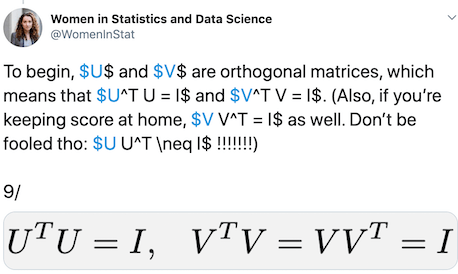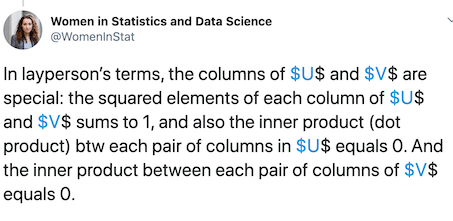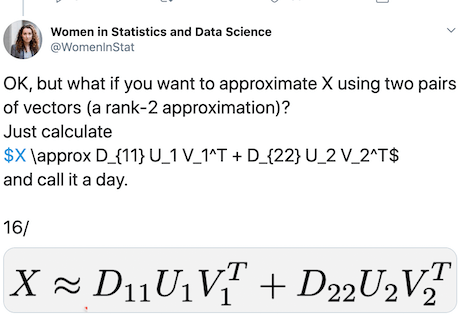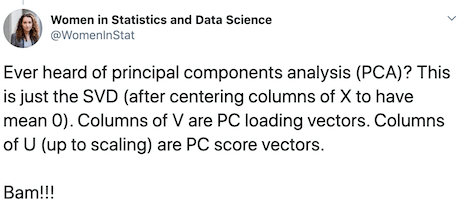## Monday, July 27, 2020

### SVD: One Matrix Decomposition to Rule Them All

One of the nice things about twitter, when you follow awesome people, is that you will come across tweets that will just blow your mind. Last week is just one such week with some fantastic and funniest tweetorials.

One of the tweetorials was from Prof. Daniela Witten for @WomenInStat. And it starts like this and beautifully explains why Singular Value Decomposition – SVD in short, is the one matrix decomposition that rules over all the others.

Being always in the #teamSVD camp, here is a blog post that tries to unpack some of the tweets with real data example using the awesome Penguins data.

Before we jump in to SVD, let us load tidyverse, the suit of R packages.

library("tidyverse")
theme_set(theme_bw(16))


We will use the Palmer Penguins data collated by Allison Horst directly from cmdlinetips.com‘s github page.

p2data <- "https://raw.githubusercontent.com/cmdlinetips/data/master/palmer_penguins.csv"

penguins_df <- read_csv(p2data)
## # A tibble: 6 x 7
##   species island bill_length_mm bill_depth_mm flipper_length_… body_mass_g sex
##   <chr>   <chr>           <dbl>         <dbl>            <dbl>       <dbl> <chr>
## 1 Adelie  Torge…           39.1          18.7              181        3750 male
## 2 Adelie  Torge…           39.5          17.4              186        3800 fema…
## 3 Adelie  Torge…           40.3          18                195        3250 fema…
## 4 Adelie  Torge…           NA            NA                 NA          NA <NA>
## 5 Adelie  Torge…           36.7          19.3              193        3450 fema…
## 6 Adelie  Torge…           39.3          20.6              190        3650 male


Let us separate the numerical data part of the penguins data from the columns with penguin information.

penguins_meta <- penguins_df %>%
drop_na() %>%
select_if(is.character)

## # A tibble: 6 x 3
##   species island    sex
##   <chr>   <chr>     <chr>


We store the numerical variables describing penguins in a new variable and use it to do SVD.

penguins <- penguins_df %>%
drop_na() %>%
select_if(is.numeric)

## # A tibble: 6 x 4
##   bill_length_mm bill_depth_mm flipper_length_mm body_mass_g
##            <dbl>         <dbl>             <dbl>       <dbl>
## 1           39.1          18.7               181        3750
## 2           39.5          17.4               186        3800
## 3           40.3          18                 195        3250
## 4           36.7          19.3               193        3450
## 5           39.3          20.6               190        3650
## 6           38.9          17.8               181        3625


### What is Singular Value Decomposition (SVD)?

We can use svd() function in R to do SVD. We provide the data matrix as input to svd() function.

# perform SVD using svd()
penguins_svd <- svd(penguins)


What happens when we apply svd() to a data matrix?

In R, we get a list of three objects corresponding to matrix decomposition, singular values d, and singular vectors u and v from svd() function.

str(penguins_svd)
## List of 3
##  $d: num [1:4] 78250.5 499.9 74 41.8 ##$ u: num [1:333, 1:4] -0.048 -0.0486 -0.0416 -0.0442 -0.0467 ...
##  $v: num [1:4, 1:4] -0.01022 -0.00389 -0.04657 -0.99886 0.1985 ...  Let us take a look at the singular values and vectors and understand their properties. For the sake of simplicity, let us give a simple name to singular values and vectors. The sigular vector U is a matrix of dimension 333 x 4, the same dimension as our data. U <- penguins_svd$u
U %>% dim()
##  333   4


And it looks like this.

U %>% head()

##             [,1]       [,2]        [,3]        [,4]
## [1,] -0.04798188 0.01338848 0.004108109  0.07663240
## [2,] -0.04862309 0.01809574 0.014438916  0.03365464
## [3,] -0.04160792 0.08855716 0.025312973 -0.01876370
## [4,] -0.04415929 0.06451464 0.067224723  0.03968621
## [5,] -0.04671088 0.04099607 0.024614580  0.08738297
## [6,] -0.04638621 0.02499507 0.006737752  0.04717352


Similarly, the singular vectors V is of the dimension 4 x 4.

V <- penguins_svd$v  And the singular vectors V looks like these. V %>% head() ## [,1] [,2] [,3] [,4] ## [1,] -0.010218185 0.19849831 -0.975661291 -0.092623187 ## [2,] -0.003891394 0.14068499 -0.065122188 0.987902673 ## [3,] -0.046569777 0.96877576 0.209389404 -0.124341735 ## [4,] -0.998855196 -0.04774608 0.000472226 0.002896004  And finally, the singular values D we get is a vector of length 4. D <- penguins_svd$d
D
##  78250.54165   499.88402    74.04578    41.84098


### What is special about these singular matrices?

The singular matrices U and V are orthogonal. What this means is if you multiply U with transpose of U, you will get an identity matrix with ones along diagonal and zeros elsewhere. This is true for the singular matrix V as well.svd_orthogonal_matrix

Let us verify the orthogonal properties of the singular matrices from the penguin data.

t(U) %*% U


We can see that diagonal elements are ones and off diagonal elements pretty close to zero.

##              [,1]         [,2]          [,3]          [,4]
## [1,] 1.000000e+00 2.038300e-16  3.816392e-17  6.126555e-16
## [2,] 2.038300e-16 1.000000e+00  2.289835e-16  2.167862e-16
## [3,] 3.816392e-17 2.289835e-16  1.000000e+00 -1.156844e-16
## [4,] 6.126555e-16 2.167862e-16 -1.156844e-16  1.000000e+00

And this is true of the singular matrix V as well.

t(V) %*% V
##               [,1]          [,2]          [,3]          [,4]
## [1,]  1.000000e+00 -4.857226e-17  1.919038e-17 -3.473784e-16
## [2,] -4.857226e-17  1.000000e+00  2.058629e-17  5.583641e-18
## [3,]  1.919038e-17  2.058629e-17  1.000000e+00 -1.406308e-17
## [4,] -3.473784e-16  5.583641e-18 -1.406308e-17  1.000000e+00


Also, this is true if you change the order of multiplication as well.

V %*% t(V)
##               [,1]         [,2]          [,3]          [,4]
## [1,]  1.000000e+00 5.551115e-17  2.255141e-17 -6.179952e-18
## [2,]  5.551115e-17 1.000000e+00  8.326673e-17  3.486794e-16
## [3,]  2.255141e-17 8.326673e-17  1.000000e+00 -1.534146e-17
## [4,] -6.179952e-18 3.486794e-16 -1.534146e-17  1.000000e+00


Another special property of the singular vectors is that orthonormal. What this means is that the squared elements of each column of U sums to 1. And the inner product (dot product) between any pair of columns in U equals to 0. And the inner product between each pair of columns of V equals 0. The same is true for V columns as well.svd_singular_vectors

Let us first check if the squared elements of each column equals 1 for U and V.

colSums(U^2)
##  1 1 1 1

colSums(V^2)
##  1 1 1 1


We see that the sum of squares for each columns of U and V is 1.

Now let us check if the column vectors are orthogonal, i.e. does the inner product of a pair U columns (and also V columns) equals to zero.

We see that the column vectors of U and V are orthogonal.

sum(V[,1]*V[,4])
##  -3.474868e-16


sum(U[,1]*U[,2])
##  1.715343e-16


Since both the properties “orthogonal” and “normality” hold true for U and V, we say that the singular vectors are orthonormal.

### Reconstructing Original data with a few singular vectors

One of the common applications of SVD is in data compression or dimensionality reduction. The original data matrix with bigger dimension can be approximated with one or few singular vectors.SVD_rank_1_approximation

Let us reconstruct an approximate version of the original data using one column vector from U and V and the first singular value. We get rank-1 approximation of the data.

D * (U[,1] %*% t(V[,1])) %>% head()
##          [,1]     [,2]     [,3]     [,4]
## [1,] 38.36528 14.61066 174.8513 3750.310
## [2,] 38.87798 14.80591 177.1879 3800.427
## [3,] 33.26880 12.66977 151.6239 3252.115
## [4,] 35.30882 13.44667 160.9213 3451.533
## [5,] 37.34901 14.22364 170.2196 3650.967
## [6,] 37.08941 14.12477 169.0365 3625.591


Let us check the top few rows from rank-1 approximate data with the original data. And we can see that, rank-1 approximation is pretty close to original data.

penguins %>% head()
## # A tibble: 6 x 4
##   bill_length_mm bill_depth_mm flipper_length_mm body_mass_g
##            <dbl>         <dbl>             <dbl>       <dbl>
## 1           39.1          18.7               181        3750
## 2           39.5          17.4               186        3800
## 3           40.3          18                 195        3250
## 4           36.7          19.3               193        3450
## 5           39.3          20.6               190        3650
## 6           38.9          17.8               181        3625


We can improve our ability to reconstruct by using more singular vectors.SVD_rank_2_approximation

In this case let us compute rank-2 approximation of data matrix using the first column singular vectors and singular values.

((D * (U[,1] %*% t(V[,1])))+ (D * (U[,2] %*% t(V[,2]))))  %>% head()

##          [,1]     [,2]     [,3]     [,4]
## [1,] 39.69377 15.55222 181.3350 3749.991
## [2,] 40.67355 16.07852 185.9512 3799.995
## [3,] 42.05598 18.89765 194.5099 3250.001
## [4,] 41.71036 17.98374 192.1642 3449.993
## [5,] 41.41689 17.10673 190.0730 3649.989
## [6,] 39.56958 15.88258 181.1410 3624.994


When we compare this to original penguins data, we can see that it is a very good approximation.

### Computing PCA from SVD

Principal component analysis is just a special case of SVD. Applying SVD on the data matrix whose columns are centered, is doing PCA. Columns of V are PC loading vectors. Columns of U are PC score vectors.PCA is a special case of SVD

Let us allply SVD on mean centered Penguins data. By mean centering, we are putting all the variables/columns in the data on the same scale. Therefore a single variable with a huge range would not dominate.

# mean centering the columns
data4pca <- apply(penguins,2,function(x){x-mean(x)})
# apply SVD
pca_by_svd <- svd(data4pca)
# get a peek at V singular vectors
pca_by_svd$v %>% head() ## [,1] [,2] [,3] [,4] ## [1,] 0.004003162 -0.31927773 -0.94126475 -0.1098470736 ## [2,] -0.001154327 0.08684753 -0.14449479 0.9856862728 ## [3,] 0.015194547 -0.94354238 0.30518986 0.1278908060 ## [4,] 0.999875876 0.01571702 -0.00103611 -0.0003657482  Let us also apply PCA on Penguins data using prcomp() function. Notice that rotation matrix from PCA is the same as V singular vectors from SVD on mean centered data. pca_obj <- prcomp(penguins) pca_obj$rotation %>% head()
##                            PC1         PC2         PC3           PC4
## bill_length_mm     0.004003162 -0.31927773 -0.94126475 -0.1098470736
## bill_depth_mm     -0.001154327  0.08684753 -0.14449479  0.9856862728
## flipper_length_mm  0.015194547 -0.94354238  0.30518986  0.1278908060
## body_mass_g        0.999875876  0.01571702 -0.00103611 -0.0003657482

t(as.matrix(penguins)) %*% as.matrix(penguins)
##                   bill_length_mm bill_depth_mm flipper_length_mm body_mass_g
## bill_length_mm          654405.7     250640.99           2960705    62493450
## bill_depth_mm           250641.0      99400.11           1143413    23798630
## flipper_length_mm      2960705.0    1143412.60          13514330   284815600
## body_mass_g           62493450.0   23798630.00         284815600  6109136250


### SVD from Scratch: A Pair of Singular Vectors at a time

What is all the more interesting is writing a function from scratch to perform SVD is relatively easy. We can write a function that computes a pair of U and V using an iterative approach. Idea is to compute the first U and V singular vectors from the data iteratively and then remove the rank-1 approximation from the data and apply the approach to compute the second U and V singular vectors.Implementing SVD from Scratch

Here is an R function that computes the first singular vectors of SVD from scrtach

svd_from_scratch <- function(data){
# initialize u and v with random numbers
u <- rnorm(nrow(data))
v <- rnorm(ncol(data))
for (iter in 1:500){
u <- data %*% v
u <-  u/sqrt(sum(u^2))
v <- t(data) %*% u
v <- v / sqrt(sum(v^2))
}
return(list(u=u,v=v))
}


svdx <- svd_from_scratch(as.matrix(penguins))

cor(U[,1],svdx$u) ## [,1] ## [1,] -1 cor(V[,1],svdx$v)
##      [,1]
## [1,]   -1


If you love the tweetorial from Prof. Daniela Witten, you would love the “An Introduction to Statistical Learning with Applications in R” co-authored by Prof. Witten. And it is freely available online.

And can’t wait to get my hands on the Second Edition of the book

The post SVD: One Matrix Decomposition to Rule Them All appeared first on Python and R Tips.

from Python and R Tips https://ift.tt/30Qw8LR
via Gabe's MusingsGabe's Musings